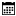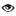#### AutoMapper

Rakesh Kumar Sutar |20 January 2021 |1372

## Introduction

C# provides a number of operators. Many of them are supported by the built-in types and allow you to perform basic operations with values of those types. Those operators include the following groups:

-Arithmetic operators
-Relational operators
-Comparison operators
-Boolean logical operators
-Bitwise and shift operators
-Assignment operator

## Arithmetic Operators

Operator Description Example
Adds two operands A B = 30
- Subtracts second operand from the first A - B = -10
* Multiplies both operands A * B = 200
/ Divides numerator by de-numerator B / A = 2
% Modulus Operator and remainder of after an integer division B % A = 0
Increment operator increases integer value by one A = 11
-- Decrement operator decreases integer value by one A-- = 9

## Relational Operators

Operator Description Example
== Checks if the values of two operands are equal or not, if yes then condition becomes true. (A == B) is not true.
!= Checks if the values of two operands are equal or not, if values are not equal then condition becomes true. (A != B) is true.
> Checks if the value of left operand is greater than the value of right operand, if yes then condition becomes true. (A > B) is not true.
< Checks if the value of left operand is less than the value of right operand, if yes then condition becomes true. (A < B) is true.
>= Checks if the value of left operand is greater than or equal to the value of right operand, if yes then condition becomes true. (A >= B) is not true.
<= Checks if the value of left operand is less than or equal to the value of right operand, if yes then condition becomes true. (A <= B) is true.

## Logical Operators

Operator Description Example
&& Called Logical AND operator. If both the operands are non zero then condition becomes true. (A && B) is false.
|| Called Logical OR Operator. If any of the two operands is non zero then condition becomes true. (A || B) is true.
! Called Logical NOT Operator. Use to reverses the logical state of its operand. If a condition is true then Logical NOT operator will make false. !(A && B) is true.

## Bitwise Operators

p q p & q p | q p ^ q
0 0 0 0 0
0 1 0 1 1
1 1 1 1 0
1 0 0 1 1

## Assignment Operators

Operator Description Example
= Simple assignment operator, Assigns values from right side operands to left side operand C = A B assigns value of A B into C
= Add AND assignment operator, It adds right operand to the left operand and assign the result to left operand C = A is equivalent to C = C A
-= Subtract AND assignment operator, It subtracts right operand from the left operand and assign the result to left operand C -= A is equivalent to C = C - A
*= Multiply AND assignment operator, It multiplies right operand with the left operand and assign the result to left operand C *= A is equivalent to C = C * A
/= Divide AND assignment operator, It divides left operand with the right operand and assign the result to left operand C /= A is equivalent to C = C / A
%= Modulus AND assignment operator, It takes modulus using two operands and assign the result to left operand C %= A is equivalent to C = C % A
<<= Left shift AND assignment operator C <<= 2 is same as C = C << 2
>>= Right shift AND assignment operator C >>= 2 is same as C = C >> 2
&= Bitwise AND assignment operator C &= 2 is same as C = C & 2
^= bitwise exclusive OR and assignment operator C ^= 2 is same as C = C ^ 2
|= bitwise inclusive OR and assignment operator C |= 2 is same as C = C | 2